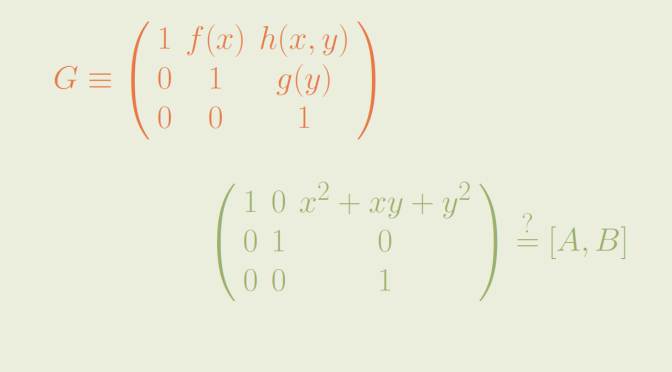# The set of all commutators in a group need not be a subgroup

I here provide a simple example of a group whose set of commutators is not a subgroup. The counterexample is due to P.J. Cassidy (1979).

### Description of the group $$G$$

Let $$k[x,y]$$ denote the ring of all polynomials in two variables over a field $$k$$, and let $$k[x]$$ and $$k[y]$$ denote the subrings of all polynomials in $$x$$ and in $$y$$ respectively. $$G$$ is the set of all upper unitriangular matrices of the form
$A=\left(\begin{array}{ccc} 1 & f(x) & h(x,y) \\ 0 & 1 & g(y) \\ 0 & 0 & 1 \end{array}\right)$ where $$f(x) \in k[x]$$, $$g(y) \in k[y]$$, and $$h(x,y) \in k[x,y]$$. The matrix $$A$$ will also be denoted $$(f,g,h)$$.
Let’s verify that $$G$$ is a group. The products of two elements $$(f,g,h)$$ and $$(f^\prime,g^\prime,h^\prime)$$ is
$\left(\begin{array}{ccc} 1 & f(x) & h(x,y) \\ 0 & 1 & g(y) \\ 0 & 0 & 1 \end{array}\right) \left(\begin{array}{ccc} 1 & f^\prime(x) & h^\prime(x,y) \\ 0 & 1 & g^\prime(y) \\ 0 & 0 & 1 \end{array}\right)$
$=\left(\begin{array}{ccc} 1 & f(x)+f^\prime(x) & h(x,y)+h^\prime(x,y)+f(x)g^\prime(y) \\ 0 & 1 & g(y)+g^\prime(y) \\ 0 & 0 & 1 \end{array}\right)$ which is an element of $$G$$. We also have:
$\left(\begin{array}{ccc} 1 & f(x) & h(x,y) \\ 0 & 1 & g(y) \\ 0 & 0 & 1 \end{array}\right)^{-1} = \left(\begin{array}{ccc} 1 & -f(x) & f(x)g(y) – h(x,y) \\ 0 & 1 & -g(y) \\ 0 & 0 & 1 \end{array}\right)$ proving that the inverse of an element of $$G$$ is also an element of $$G$$.

### The commutator subgroup of $$G$$ is the set of elements $$(0,0,h)$$ where $$h \in k[x,y]$$

Let’s remind that the commutator subgroup of a group is the subgroup generated by all the commutators of the group. For elements $$a$$ and $$b$$ of a group, the commutator of $$a$$ and $$b$$ is $$[a,b]=a^{-1}b^{-1}ab$$. The commutator subgroup of $$G$$, also called the derived subgroup is denoted $$G^\prime$$. One can verify that the commutator of $$(f,g,h), \ (f^\prime,g^\prime,h^\prime) \in G$$ is
$[(f,g,h),(f^\prime,g^\prime,h^\prime)]= \left(\begin{array}{ccc} 1 & 0 & f(x)g^\prime(y)-f^\prime(x)g(y) \\ 0 & 1 & 0 \\ 0 & 0 & 1 \end{array}\right)$ which is an element $$(0,0,h(x,y))$$ with $$h(x,y)=f(x)g^\prime(y)-f^\prime(x)g(y) \in k[x,y]$$. Conversely, we have to prove that for $$\displaystyle h(x,y) \in k[x,y]$$, $$(0,0,h(x,y))$$ belongs to $$G^\prime$$. When $$h(x,y)=x^ky^l$$ is a monomial, we derive from above the equality $$(0,0,x^ky^l)=[(x^k,0,0)(0,y^l,0)]$$ which proves that $$(0,0,x^ky^l)$$ is a commutator. The following equality
$\left(\begin{array}{ccc} 1 & 0 & a \\ 0 & 1 & 0 \\ 0 & 0 & 1 \end{array}\right) \left(\begin{array}{ccc} 1 & 0 & b \\ 0 & 1 & 0 \\ 0 & 0 & 1 \end{array}\right)= \left(\begin{array}{ccc} 1 & 0 & a+b \\ 0 & 1 & 0 \\ 0 & 0 & 1 \end{array}\right)$ holds. Hence we get
$(0,0,h)=\prod_{i,j}[(a_{ij}x^i,0,0),(0,y^j,0)]$ for any $$\displaystyle h(x,y)=\sum_{i,j} a_{ij}x^iy^j \in k[x,y]$$. We can finally conclude
$G^\prime=\{(0,0,h(x,y)) | \ h(x,y) \in k[x,y]\}$

### $$(0,0,x^2+xy+y^2)$$ is not a commutator

If $$(0,0,x^2+xy+y^2)$$ is a commutator, then there are polynomials $$f(x),f^\prime(x) \in k[x]$$ and $$g(y),g^\prime(y) \in k[y]$$ with
$x^2+xy+y^2=f(x)g^\prime(y)-f^\prime(x)g(y)$ If $$f(x) = \sum b_i x^i$$ and $$f^\prime(x) = \sum b_i^\prime x^i$$, then there are equations:
$\left\{\begin{array}{lll} b_0 g^\prime(y) – b_0^\prime g(y) & = & y^2 \\ b_1 g^\prime(y) – b_1^\prime g(y) & = & y \\ b_2 g^\prime(y) – b_2^\prime g(y) & = & 1 \end{array}\right.$ which cannot be as this implies that the two polynomials $$g(y),g^\prime(y)$$ generate the three linearly independent polynomials $$\{1,y,y^2\}$$.
Based on this result, we derive that the product of the two commutators $$[(x^2,-y,0)(x,1,0)]=(0,0,x^2+xy)$$ and $$[(0,y^2,0)(0,0,0)]=(0,0,y^2)$$ which is equal to $$(0,0,x^2+xy+y^2)$$ is not a commutator.

### Case of finite groups

The group $$G$$ is infinite. It is also possible to find a finite group whose set of commutator is not a subgroup. I might come back on this topic later on. The smallest group having this feature is of order $$96$$.